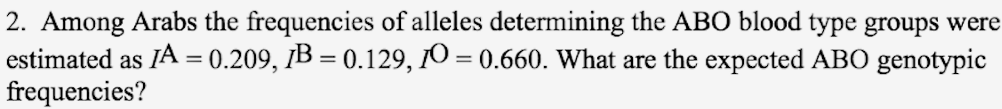# Problem: Among Arabs, the frequencies of alleles determining the ABO blood type groups were estimated as IA = 0.209, IB = 0.129, IO = 0.660. What are the expected genotypic frequencies?

###### FREE Expert Solution

With the assumption that the population under study is in Hardy-Weinberg equilibrium, the genotypic frequencies can be computed as follows:###### Problem Details

Among Arabs, the frequencies of alleles determining the ABO blood type groups were estimated as IA = 0.209, IB = 0.129, IO = 0.660. What are the expected genotypic frequencies?